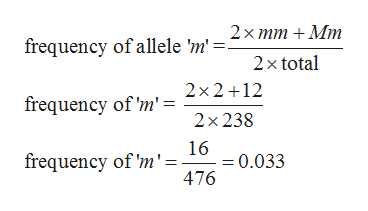# A population of 238 cichlid fish are sampled and genotyped. Below is the distribution of genotypes. Calculate the probability that the recessive allele will become fixed due to drift alone. GenotypeNumber of individualsMM224Mm12mm2

Question
1. A population of 238 cichlid fish are sampled and genotyped. Below is the distribution of genotypes. Calculate the probability that the recessive allele will become fixed due to drift alone.

 Genotype Number of individuals MM 224 Mm 12 mm 2

check_circleExpert Solution
Step 1

In a given population of 238 cichlid fish, the distribution of genotypes is:

MM (homozygous dominant) = 224

Mm (heterozygous) = 12

Mm (homozygous recessive) = 2

Step 2

The probability at any time that an allele will ultimately become fixed is simply its frequency in the population. So we have to calculate the frequency of the recessive allele. That means allele ‘m’.help_outlineImage Transcriptionclose2х тm + Mmт frequency of allele 'm'. 2x total 2x2 12 frequency of 'm' = 2х 238 16 = 0.033 476 frequency of 'm'= fullscreen
Step 3

found frequency of allele ‘m’=0.033

So the probability of recessive allele ‘m’ is also 0.033 or 3.3%

That means alle...

### Want to see the full answer?

See Solution

#### Want to see this answer and more?

Solutions are written by subject experts who are available 24/7. Questions are typically answered within 1 hour*

See Solution
*Response times may vary by subject and question
Tagged in

### Genetics